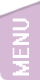[精讚] [會員登入]
2525

### [PERL] 09-迴圈

perl的迴圈

#### 此文完整連結http://n.sfs.tw/11683

##### 2017-08-23 13:21:12 By 張○○while

do...while

do...until

foreach

for

## while

while( 符合條件 ){ 處理 }

$a=0; while($a<10){
print "."; #共印出10個點
$a ++; } ## do...while 寫法 do{ 處理 }while( 符合條件 ); 和while不一樣是一開始一定會執行一次，然後再判斷會不會繼續執行迴圈。注意最後面的分號。 $a=0;
do{
print ".";
$a ++; }while($a<10);

## do...until

do{ 處理 } until( 不符合條件 );

$a=0; do{ print ".";$a ++;
}until($a>=10); ## for 寫法 for( 變數初始值;符合條件;運算 ){ 處理 } for 項目 ( 範圍 ){ 處理 } #### 輸出數字 1-10 for($ii=1;$ii<=10;$ii++){
print $ii . "\n"; }  這樣寫太麻煩了，PERL中可以用範圍運算子來作 for$ii (1..10){
print $ii . "\n"; }  再懶點的把$ii也偷懶的省略掉，但省略掉後要怎麼代表$ii呢？ PERL就用一個特殊變數$_來代替，這就是「預設變數」。在 09- 雜湊這個單元有先提過，未來只要看到 $_ 也不必大驚小怪了。改寫後的結果是 for (1..9){ print$_ . "\n";
}


#### 輸出 A-Z 26個字母

PERL還有一些神奇的技巧，要用迴圈印出大寫英文字母怎麼做？你不必去翻ASCII然後把數字轉成字元。只要這樣就行了：

for ('A'..'Z'){
print $_ . "\n"; }  ## foreach 寫法 foreach 項目 ( 陣列或清單 ){ 處理 } #### 使用foreach列出陣列內容 @name = qw (John Mary Robinson 王大明 李小美); foreach$one (@name){
print $one. "\n"; }  結果 John Mary Robinson 王大明 李小美 上面的$one 就是陣列中的項目，在foreach中依序被叫出，如前所述，我們把它省略掉：

@name = qw (John Mary Robinson 王大明 李小美);
foreach (@name){
for($jj=2;$jj<10;$jj++){ last if$ii==$jj; last CYCLE1 if$ii*$jj>50; print "$ii*$jj=".$ii*$jj. "\t"; } print "\n"; }  第1行 這個迴圈標記名稱 CYCLE1 第4行 中如果相乘大於50，last到標籤 CYCLE1 結果 3*2=6 4*2=8 4*3=12 5*2=10 5*3=15 5*4=20 6*2=12 6*3=18 6*4=24 6*5=30 7*2=14 7*3=21 7*4=28 7*5=35 7*6=42 8*2=16 8*3=24 8*4=32 8*5=40 8*6=48 #### redo 這個項目重做一次迴圈，這個操作只會進行迴圈中{ }裡面的操作，不會再去評估是否符合條件(如果有設條件的話，例如while(條件)) 我舉一個範例，假設Mary, Steve, John三個人要隨機抽數字，沒抽中就重抽，直到中為止，列出大家要抽中的需要的次數 @name= ('Mary', 'Steve', 'John');$prizenum=33;
$pick=0; foreach( @name){$yournum=int rand() *100 ;
$pick++; redo if$prizenum != $yournum; print "$_ 抽了 $pick 次得獎\n";$pick= 0;
}


Mary 抽了 68 次得獎
Steve 抽了 13 次得獎
John 抽了 20 次得獎

END

### 你可能感興趣的文章

Perl 的列舉寫法(map list) 使用列舉的寫法來設定陣列，非常的強大

[PERL] 14-進階比對 #1--取回比對內容 perl的正規表達式進階比對

PERL 正規表達式會用到的符號 PERL在比對時常常會用到符號整理

[PERL] 前置if和後置if (if修飾子)的差別 perl的if可以拿來作為後置修飾子使用，此外還有一個unless像是if的相反，分析他們的差異

[PERL] 21- 使用資料庫 Perl 連Mysql

PERL一次讀取檔案所有內容的方法(\$/) perl可以使用slurp mode一次讀完檔案所有內容的方法及一些範例

[PERL] 08-陣列 #2 --操作 更多perl陣列的操作，如拆開、黏合、取出、加入等等

[PERL] 18-套件及模組 套件和模組入門

[無留言]

### 隨機好文

[MAC] 截取螢幕畫面的方法 截取螢幕畫面的方法，在MAC中叫作螢幕快照，英文是screenshot

SELinux 常用指令和檔案 在Redhat系列中，Centos5以後加入了selinux，他並沒有這麼可怕，不必每次看到Selinux ，就想把他

[JAVA] JWS, JWT, JWE, JOSE是什麼？ [JAVA] JWS, JWT, JWE, JOSE是什麼？非常的複雜，儘量來搞清楚..

[大型機台] 熱血高校躲避球 多少少年時光歲月耗在這個遊戲上，二十幾年前的那個時光，唯一想做的事就是把吃飯錢省下來拿去打一場五塊錢的電動，就算是沒錢也